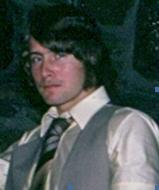Enter the content which will be displayed in sticky bar

# MemberDr. Gerard Coutu
local time: 2020-07-12 18:43 (-04:00 DST)
Dr. Gerard Coutu (Abstracts)
Titles
• [Updated 3 years ago]
• [Updated 9 years ago]
• [Updated 9 years ago]
• [Updated 9 years ago]
• [Updated 9 years ago]
Abstracts Details
• [Updated 3 years ago]

The Hering furnace and overhead welding have previously been analyzed from the po1ot of view of the currently taught classical equation and the new Gaussian equation of Moon, Spencer, et al for the force between moving charges. This paper extends this analysis to the Weber equation of 1846. Conclusions are drawn on the validity of each of these three electrodynamic equations.

• [Updated 9 years ago]

The paper presents an experimental verification of the validity of the new Gaussian equation for the force between moving charges. Equations for the force between current elements which are derived from the Weber equation, the classical equation and the new Gaussian equation are presented. These equations are applied to the Hering furnace. The operation of the Hering furnace is consistent with the new Gaussian equation for the force between moving charges. There are difficulties with the Weber equation and the classical equation predicts that a furnace which has been used In industry for nearly a century cannot possibly operate.

• [Updated 9 years ago]

The paper presents a second experimental verification of the validity of the new Gaussian equation for the force between moving charges. Three equations for the force between moving charges are applied to the problem of overhead welding: the Weber equation, the classical equation and the new Gaussian equation. From the new Gaussian equation, it is found that large electromagnetic forces are present which hold the weld bead in place. According to the classical equation, these forces do not exist and overhead welding is impossible. According to the Weber equation, the weld bead is repelled by a large electromagnetic force!

• [Updated 9 years ago]

• [Updated 9 years ago]